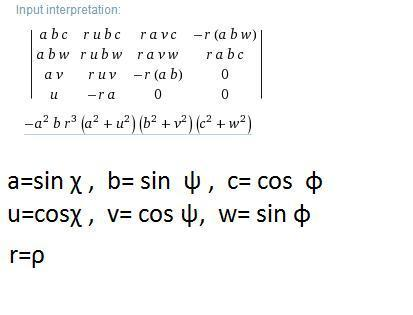# Sign of Levi-Civita Symbol in spherical coordinates

• cedricyu803
In summary, the sign of the Levi-Civita symbol is changed when you go from Cartesian coordinates to spherical coordinates. However, the Jacobian is still positive, as long as you calculate it correctly.

#### cedricyu803

Hi, I am going through the derivation of an instanton solution (n=1) in Srednicki Chp. 93.

Specifically, I went through eqn.s 93.29-93.38.
However the sign of the Levi-Civita Symbol is bugging me:

It says that in 4D Euclidean space,
$$\epsilon^{1234}=+1$$ in Cartesian coordinates
implies
$$\epsilon^{\rho \chi \psi \phi}=-1$$
in spherical coordinates
below eqn 93.17, by computing the Jacobian of coordinate change.
But from my calculation, the Jacobian is positive, simply
$$\rho^3 sin^2 \chi sin\psi$$

In the simplest case, e.g. 2D or 3D, it seems to me that the Levi-Civita symbol doesn't change sign either under this coordinate transformation.
Can anyone explain why the sign of the Levi-Civita symbol is changed?

Thanks!

$J = \begin{pmatrix} \sin \chi ~ \sin \psi ~ \cos \phi & \rho \cos \chi ~ \sin \psi ~ \cos \phi & \rho \sin \chi ~ \cos \psi ~ \cos \phi& - \rho \sin \chi ~ \sin \psi ~ \sin \phi \\ \sin \chi~ \sin \psi~ \sin \phi & \rho \cos \chi~ \sin \psi~ \sin \phi & \rho \sin \chi~ \cos \psi~ \sin \phi & \rho \sin \chi~ \sin \psi~ \cos \phi \\ \sin \chi ~ \cos \psi & \rho \cos \chi ~ \cos \psi & - \rho \sin \chi ~ \sin \psi & 0 \\ \cos \chi & -\rho \sin \chi & 0 & 0 \end{pmatrix}$

$- \rho^3 \sin \psi ~ \sin^2 \chi$Last edited:
•cedricyu803
Ahh right, now I got it. Thanks a lot

Followup question:
I am struggling to move from eq. 93.16 to 93.17.
Now that

$$n=\frac{1}{16 \pi^2} \int d^4x Tr(F_{\mu \nu}\tilde{F}^{\mu \nu})$$
$$=\frac{1}{16 \pi^2} \int d^4x \partial _\mu Tr(\epsilon^{\mu \nu \sigma \tau} (A_\nu F_{\sigma \tau}+\frac{2i}{3} A_\nu A_\sigma A_\tau))$$
$$\equiv \frac{1}{16 \pi^2} \int d^4x \partial _\mu Tr(\epsilon^{\mu \nu \sigma \tau} K_{\nu \sigma \tau})$$
$$n=\frac{1}{16 \pi^2} \int dS_\mu Tr(\epsilon^{\mu \nu \sigma \tau} K_{\nu \sigma \tau})$$
This is eq. 93.17.
So,
$$n=\frac{1}{16 \pi^2} \int (abs|J|) d\rho d\chi d\psi d\phi (\frac{1}{-(abs|J|)} \epsilon^{\delta \alpha \beta \gamma}) \partial_\delta Tr(K_{ \alpha \beta \gamma})$$
$$=\frac{-1}{16 \pi^2} \int d\rho d\chi d\psi d\phi ~ \epsilon^{\delta \alpha \beta \gamma} \partial_\delta Tr(K_{ \alpha \beta \gamma})$$
where $$\alpha, \beta, \gamma, \delta$$ are spherical coordinates
$$abs(|J|)\equiv + \rho^3 \sin \psi ~ \sin^2 \chi$$
But how precisely should I do the final step of changing the volume integral to surface integral to get 93.16?
I know by divergence theorem, but it seems to me that
from
$$\epsilon^{\delta \alpha \beta \gamma}$$
$$\epsilon^{\rho \chi \psi \phi}=-1$$
to
$$\epsilon^{\alpha \beta \gamma}$$
$$\epsilon^{\chi \psi \phi}=+1$$

Eq. 93.16 is:
$$n=\frac{-1}{16 \pi^2} \int d\chi d\psi d\phi ~ \epsilon^{\alpha \beta \gamma} Tr(K_{ \alpha \beta \gamma})$$

the minus sign from the previous step gets canceled out, giving no minus sign in the end (93.16).
Can you illustrate how to do it?
Thanks

Last edited:
•cedricyu803

## 1. What is the Sign of Levi-Civita Symbol in spherical coordinates?

The Sign of Levi-Civita Symbol in spherical coordinates is a mathematical concept used to represent the orientation of a coordinate system in three-dimensional space. It is denoted by the symbol εijk, where i, j, and k are indices ranging from 1 to 3, and it takes on a value of either 1, -1, or 0 depending on the order of the indices. This symbol is often used in vector calculus and differential geometry to simplify calculations involving cross products and determinants.

## 2. How is the Sign of Levi-Civita Symbol defined?

The Sign of Levi-Civita Symbol is defined as follows: εijk = 1 if the indices ijk form an even permutation of 123, -1 if they form an odd permutation, and 0 if any two indices are equal. In spherical coordinates, the indices correspond to the three angular variables φ, θ, and ρ, respectively. Therefore, the sign of εijk depends on the order in which these angles are arranged.

## 3. What is the significance of the Sign of Levi-Civita Symbol in spherical coordinates?

The Sign of Levi-Civita Symbol is significant in spherical coordinates because it helps determine the direction of a vector or a coordinate system in three dimensions. It is also used in various mathematical operations, such as calculating the gradient, curl, and divergence of a vector field. Additionally, it has applications in physics, particularly in the study of electromagnetic fields and fluid mechanics.

## 4. How does the Sign of Levi-Civita Symbol change with different coordinate systems?

The Sign of Levi-Civita Symbol is dependent on the orientation of the coordinate system, and therefore, it changes with different coordinate systems. In spherical coordinates, the sign differs based on the order of the angular variables, as mentioned earlier. In other coordinate systems, such as cylindrical or Cartesian coordinates, the sign may also vary depending on the arrangement of the indices.

## 5. Can the Sign of Levi-Civita Symbol be negative?

Yes, the Sign of Levi-Civita Symbol can be negative. As stated earlier, it takes on a value of -1 when the indices form an odd permutation. In spherical coordinates, this occurs when the angular variables are arranged in a clockwise direction instead of counterclockwise. However, it is important to note that the sign itself is not a negative number, but rather an indicator of the orientation or direction of the coordinate system.